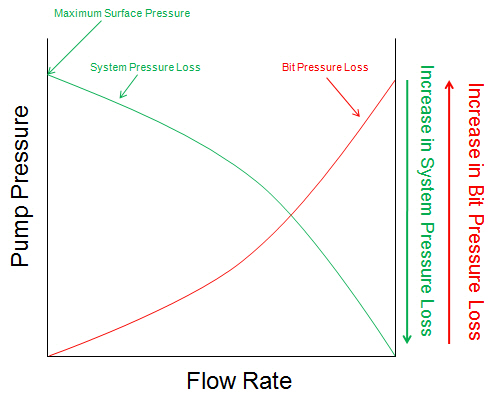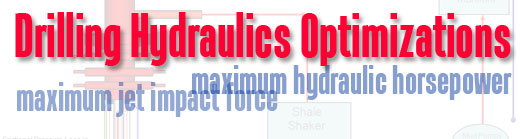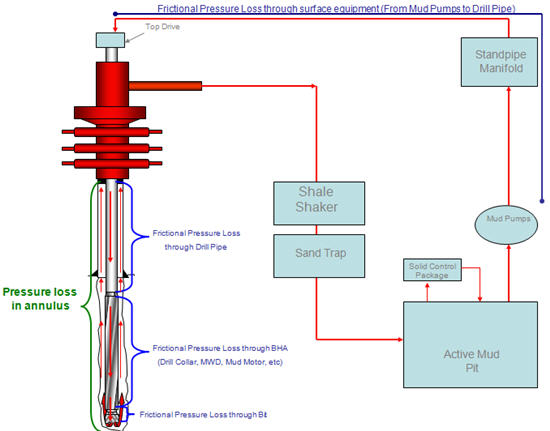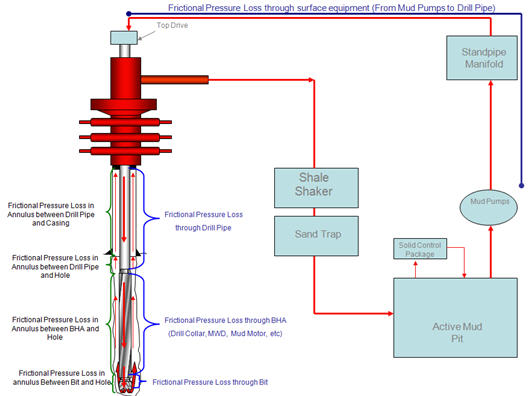## Determine The Optimized Points for Drilling Hydraulics

The design of hydraulic optimization begins with downhole component and an analysis of pressure losses as a function of flow rate.

Before I will explain you about the pressure and flow rate curve, I would like to get the following term across. The surface pressure (pump pressure) is equal to the system pressure loss and the pressure loss across the bit.

P surface = P system + P bit

The curve below demonstrates a system pressure loss curve and a pressure loss across the bit as Y-axis and a flow rate as X-axis. The system pressure loss curve starts at the maximum surface pressure and is drawn invert with the bit pressure loss curve (see the curve below more understanding).## Drilling Hydraulics Optimization

For drilling hydraulics optimization, there are two ways which is the maximum jet impact force and the maximum hydraulic horsepower used to indicate good hole cleaning.The Maximum Jet Impact Force

The concept of this method is based on the concept that the best condition to clean the well is when the force provided by the jets is the maximum. Based on the mathematical solution, this condition is occurred when bit nozzles and circulation rate are properly selected to create pressure drop across the bit which is equal to 48% of total pump pressure.

For example, if the maximum expected pressure is 3,000 psi, you need to select the flow rate and the bit nozzles which will generate 1,440 psi (48% of 3,000 psi) pressure drop across the bit and leave 1560 psi (52% of 3,000 psi) for the system pressure loss.

The Maximum Hydraulic Horsepower

The concept of this method is based on the concept that the best condition to clean the well is when the hydraulic horsepower at the bit is the maximum. Based on the mathematical solution, this condition is occurred when bit nozzles and circulation rate are properly selected to create pressure drop across the bit which is equal to 65% of total pump pressure.

For example, if the maximum expected pressure is 3,000 psi, you need to select the flow rate and the bit nozzles which will generate 1,950 psi (65% of 3,000 psi) pressure drop across the bit and leave 1050 psi (35% of 3,000 psi) for the system pressure loss. Continue reading

## Pressure Loss through the Drilling System

The pressure reading that you see on surface (pump pressure) is the total pressure used to move drilling mud through the system. The total pressure is a summation of pressure loss in all sections of the system. From the definition, we can write into a mathematical relationship like this.

Pump Pressure = P surface equipment + P drill pipe + P drill collar + P down hole tools + P bit + P annulus

The illustration below demonstrates the pressure loss in the drilling system.Ideally, you want the maximum pressure drop at the bit and little pressure loss from the rest of the system.

Reference:Drilling Hydraulic Books

## Introduction to Drilling Hydraulics

Drilling hydraulics affect directly drilling performance and this topic will focus on the basic principle of the drilling hydraulics.

Circulation System on Drilling Rigs

Typically, the rig circulation system is composed of mud pumps which deliver drilling mud from mud pits to hoses and pipes down to the bottom of the hole. Drilling mud is moved through a bit into the annulus and is finally returned back to solid control equipment and the mud pits (you can see from the following diagram).Frictional Pressure Loss

Frictional Pressure Loss is pressure loss created when drilling mud flows through a flow path. Frictional pressure loss affects on pump pressure because higher frictional pressure loss, higher pump pressure is required to maintain the pump rate.

The following parameters affecting frictional pressure loss are as follows:

• Inner diameter of drill pipe, drill collar and heavy weight drill pipe

• Down hole tool as a mud motor, a rotary steerible tool, a MWD/LWD, etc

• Length of drill pipe, drill collar and heavy weight drill pipe

• Drilling fluid properties – mud weight, mud rheology (PV/YP)

• Hole size

• Length of the well

• Inner diameter of the previous casing string

• Flow area of the bit

• Flow rate

For more understanding, the diagram below demonstrates the frictional pressure loss during drilling operation.